## Want to keep learning?

This content is taken from the Raspberry Pi Foundation & National Centre for Computing Education's online course, Representing Data with Images and Sound: Bringing Data to Life. Join the course to learn more.
3.5

## Raspberry Pi Foundation

Skip to 0 minutes and 2 seconds You can convert analogue sounds into digital sounds by a process called sampling. This involves taking lots of individual measurements, which can approximate the form of the entire sound wave. Let’s look at how this would work for a simple sound wave. In order to represent how the signal changes over time, samples are taken at equally-spaced intervals. The number of samples taken each second is known as the sampling rate, which is measured in kilohertz. 1 kilohertz equals 1,000 samples per second. Common sampling rates are 44.1, 48, or 96 kilohertz. So a sampling rate of 44.1 kilohertz means that we take 44,100 samples per second.

Skip to 0 minutes and 51 seconds Now, this many samples are needed to make sure that we capture the entire frequency range of human hearing. But how is this data stored? Now, this vertical line represents one of the times a sample is taken. The height of the signal represents the voltage from the microphone. But you can’t simply store that data, because the analogue signal is continuous. In theory, no number of bits is enough to store that data accurately. Instead, a sampling resolution is chosen. Now, this determines how accurately the signal level is represented at this time. For example, 3 bits can represent eight different levels. Now when the sample is taken, the level the signal is closest to is recorded.

Skip to 1 minute and 40 seconds Now, this method of taking a point on a continuous scale and choosing a value for that point is called quantization. Even though there is a difference between the measured value and the stored value, using more bits means that we can increase the sampling resolution and get closer to the original signal. 16 bits of data gives a sample resolution of 65,536 levels. But using 24 bits increases that resolution to over 16 million different levels. Quantization is performed each time the signal is sampled, generating a series of binary numbers. Now, these binary numbers represent the height of the wave at different times. This binary representation can then be edited, modified, or used to reproduce the sound.

# Sampling

As I described in the previous step, a computer records sound by converting an analogue electrical signal into a digital signal. This involves taking lots of individual measurements to approximate the form of the entire sound wave; this process is called sampling.

Let’s look at how this works for the electrical signal produced by a very simple sound wave, represented as a sine wave of the voltage change over time.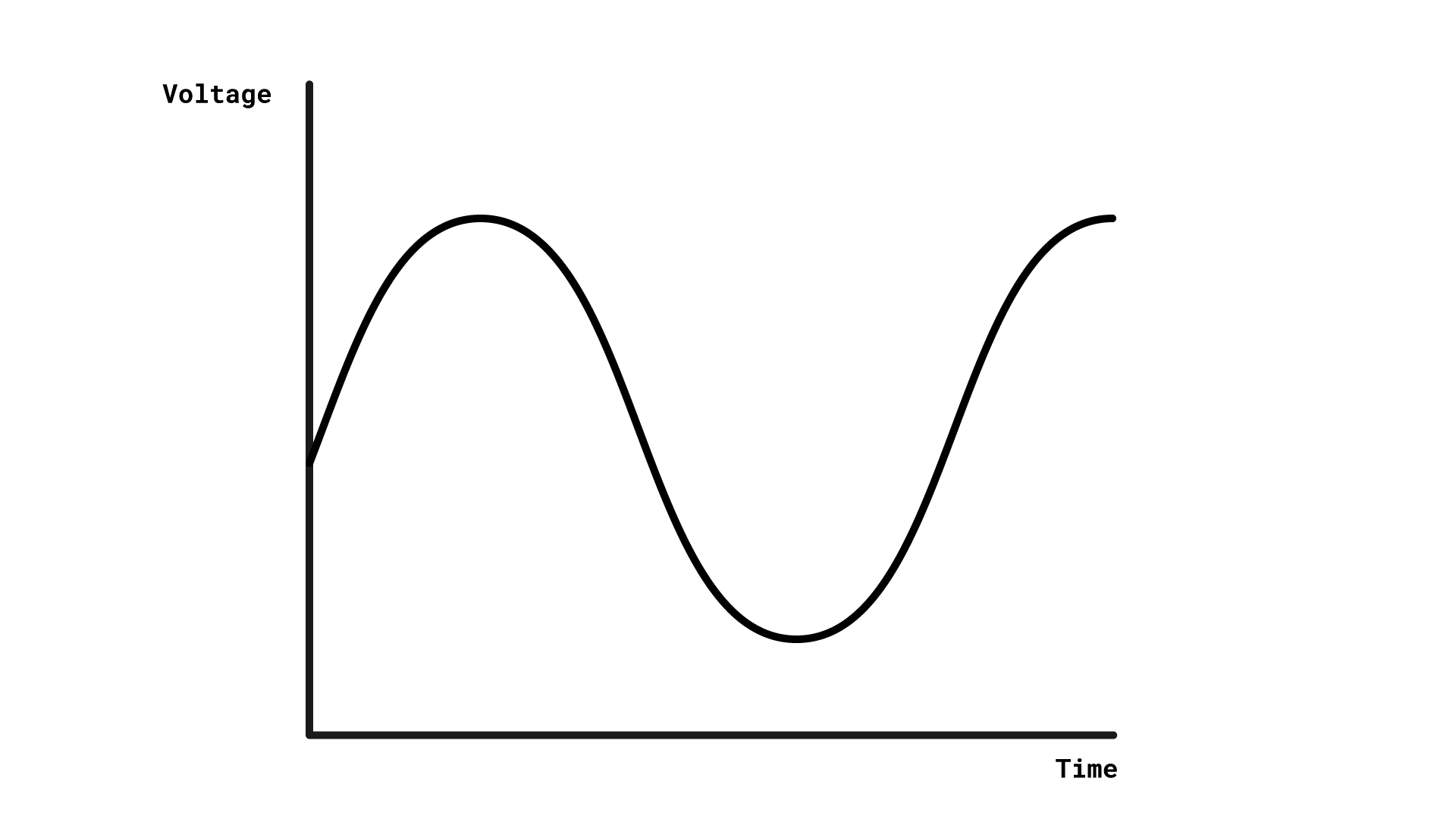## Sample rate

The computer samples the microphone’s electrical signal at lots of different time points, with the same interval between each time point and the next.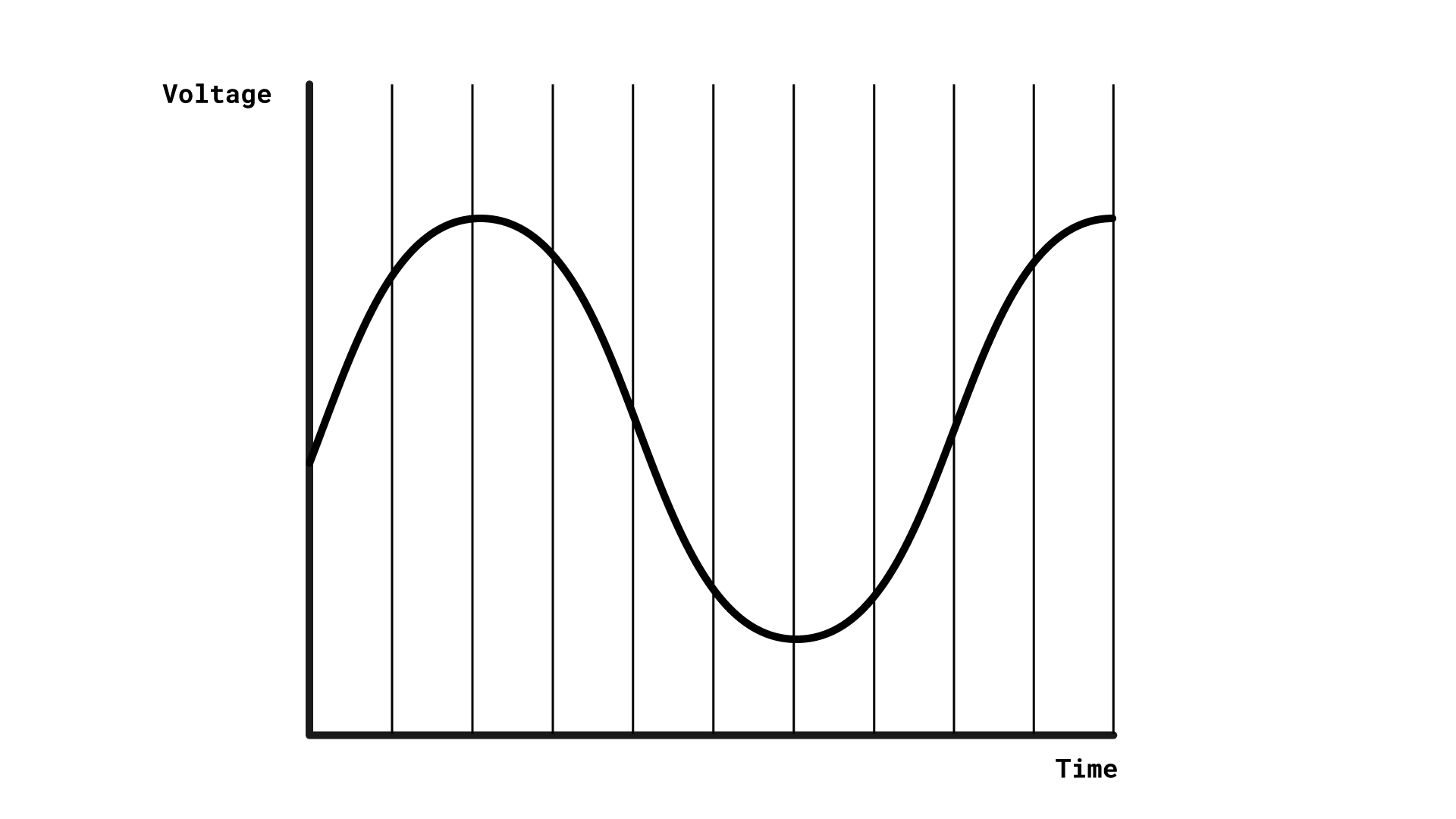The number of samples the computer takes per second is known as the sample rate. Common sample rates are 44.1 kHz, 48 kHz, and 96 kHz. kHz stands for kilohertz, or 1000 samples per second, so 44.1 kHz represents 44100 samples per second. This many samples are needed to capture the full complexity of sound waves.

## Sample resolution

Let’s look at one sample the computer takes: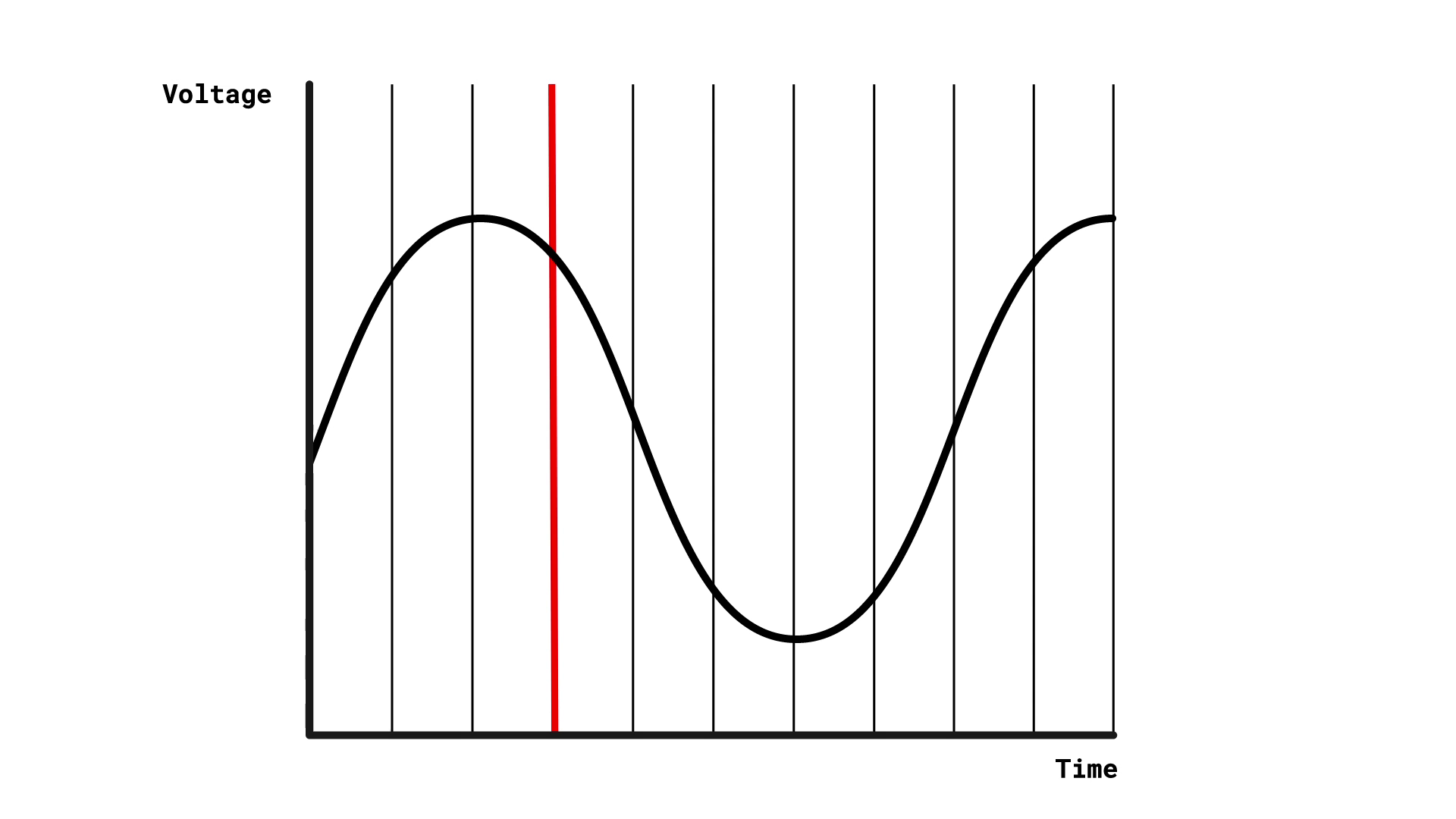You might think the computer could just read the height of the wave (which represents the electrical signal from the microphone) and store that value, but there’s a problem with that: the electrical signal is analogue, meaning it’s continuous. This means that no number of bits is enough to store the value entirely accurately. Instead, the computer has to set a sample resolution, which determines how accurately the computer represents the strength of the electrical signal.

The sample resolution is dictated by the number of bits the computer uses to store a sample value. For example, if the computer uses three bits, it can represent eight different levels of sample value; what the computer actually stores is the level that the analogue signal is closest to: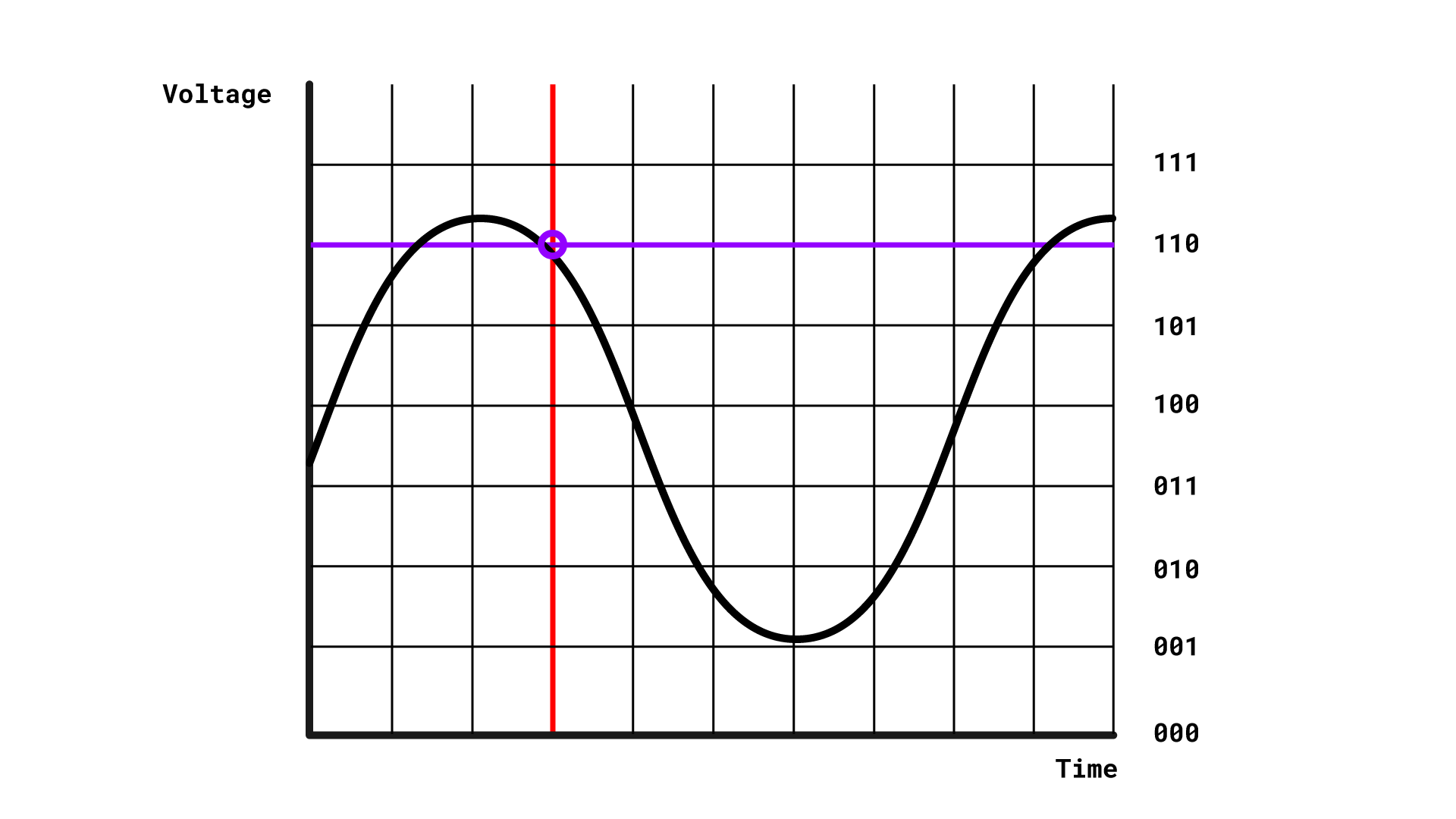Representing a point on a continuous scale with a discrete value is known as quantisation. Although there is a difference between the measured value and the stored value, a high enough sample resolution (using more bits) lets the computer get very close to the actual signal level. It’s quite common to use 16 or 24 bits, allowing the computer to represent 65536 or 16777216 different levels.

Quantisation affects sound recordings in ways that you’ve probably encountered: it can cause distortions. The sample resolution that is set for a recording process determines a maximum quantisation level. If the volume of the sound that is being recorded goes above this level, the signal becomes “clipped”, which means the recording will sound distorted.

This concept of sample resolution might remind you of something you learned about when we discussed image files in Week 2: bit depth. We defined this as the storage space each pixel needs to represent the available range of different colours; more shades of colours produce a more detailed image. Sample resolution is the matching concept for sound files: a higher sample resolution produces a more detailed sound recording. This is why sample resolution is also called audio bit depth.

## Digital storage of sound

The computer performs quantisation each time it samples the signal from the microphone, and by repeating this process, it produces a series of binary numbers that represent the height of the wave at many different time points.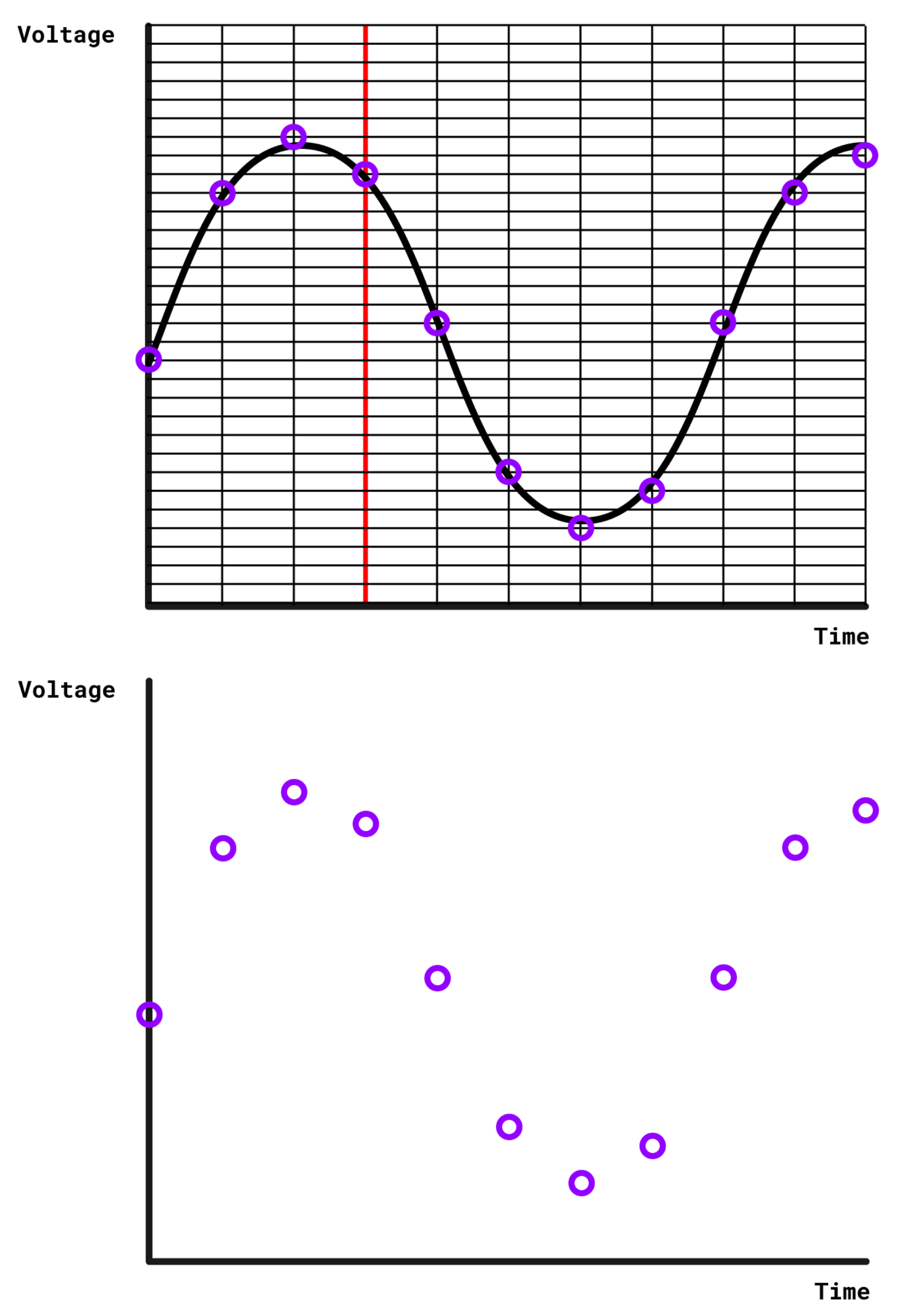The computer stores stores this binary representation as a file and can use it to edit, modify, or reproduce the recorded sound.